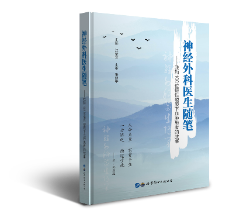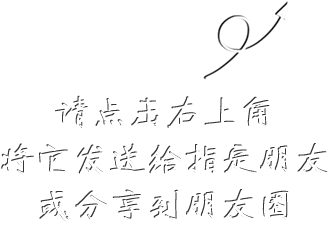998# 神经外科医生随笔——我和100位慢性硬膜下血肿患者的故事

## ¥ 140.00200.00现货已售出1件

慢性硬膜下血肿是一种多发于老年人，且在我国发病率越来越高的一种神经外科常见病，越来越引起人们重视。天津医科大学总医院神经外科教授江荣才，在其学术导师、前任中华医学会神经外科分会主任委员张建宁教授指导下持续开展阿托伐他汀钙治疗慢性硬膜下血肿新疗法的临床研究，迄今已经治愈多达400余例患者，协助张建宁教授创造了第一个有I级循证医学证据支持且被证实安全有效的治疗慢性硬膜下血肿的新疗法，获得了2020年天津市科技进步特等奖，目前正在海内外得到广泛应用。江荣才教授痛感患者和医生对该病特点还认识不足，诊疗效率还不令人满意，遂将其中100多例诊疗过程曲折、发病原因少见并都有完整临床和影像学资料的病例摘取出来，以叙事医学的风格进行讲述，清晰而全面地介绍了这个神经外科常见病的发病特点，把一个神经外科常见疾病的特点在娓娓道来的看病故事中印刻到读者的内心。同时，由于这个新疗法还在推广中，他又融进了一个神经外科主诊医生对疾病诊疗、新疗法研发、老年化带来的家庭社会问题、患者就医中遇到的各色困难等种种问题的深刻思考，附录了张建宁教授团队开发该新疗法的年谱资料和其他研究成果，使该书成为目前国内唯一一本专论慢性硬膜下血肿临床诊疗又兼具叙述医学性质的临床专著。

• 删除
• 删除
• 删除
• 删除
• 删除
• 删除
• 删除
• 删除
• 删除
• 删除
• 删除
• 删除
• 删除
• 删除
• 删除
• 删除
• 删除
• 删除
• 删除
• 删除
• 删除
• 删除
• 删除
• 删除
• 删除
• 删除
• 删除
• 删除
• 删除
• 删除
• 删除
• 删除
• 删除
• 删除
• 删除
• 删除
• 删除
• 删除
• 删除
• 删除
• 删除
• 删除
• 删除
• 删除
• 删除
• 删除
• 删除
• 删除
• 删除
• 删除
• 删除
• 删除
• 删除
• 删除
• 删除
• 删除
• 删除
• 删除
• 删除
• 删除
• 删除
• 删除
• 删除
• 删除
• 删除
• 删除
• 删除
• 删除
• 删除
• 删除
• 删除
• 删除
• 删除
• 删除
• 删除
• 删除
• 删除
• 删除
• 删除
• 删除
• 删除
• 删除
• 删除
• 删除
• 删除
• 删除
• 删除
• 删除
• 删除
• 删除
• 删除
• 删除
• 删除
• 删除
• 删除
• 删除
• 删除
• 删除
• 删除
• 删除
• 删除
• 删除
• 删除
• 删除
• 删除
• 删除
• 删除
• 删除
• 删除
• 删除
• 删除
• 删除
• 删除
• 删除
• 删除
• 删除
• 删除
• 删除
• 删除
• 删除
• 删除
• 删除
• 删除
• 删除
• 删除
• 删除
• 删除
• 删除
• 删除
• 删除
• 删除
• 删除
• 删除
• 删除
• 删除
• 删除
• 删除
• 删除
• 删除
• 删除
• 删除
• 删除
• 删除
• 删除
• 删除
• 删除
• 删除
• 删除
• 删除
• 删除
• 删除
• 删除
• 删除
• 删除
• 删除
• 删除
• 删除